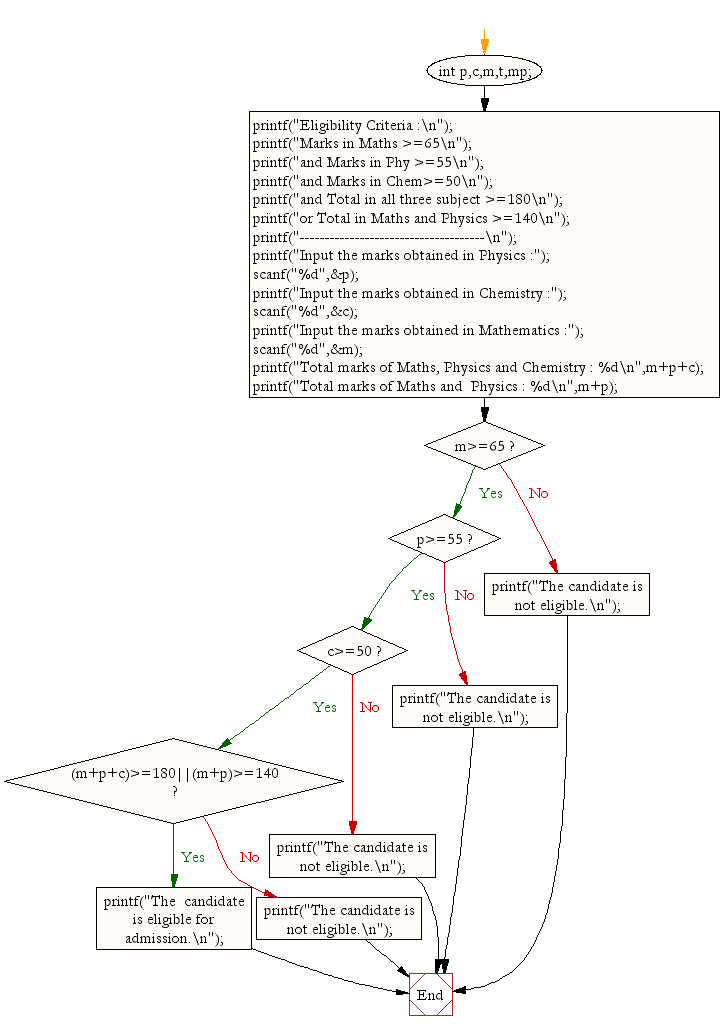﻿ C exercises: Find eligibility for admission using Nested If Statement - w3resource# C Exercises: Find eligibility for admission using Nested If Statement

## C Conditional Statement: Exercise-10 with Solution

Write a C program to find the eligibility of admission for a professional course based on the following criteria:
Marks in Maths >=65
Marks in Phy >=55
Marks in Chem>=50
Total in all three subject >=180
or
Total in Math and Physics >=140

Sample Solution:

C Code:

``````#include <stdio.h>
void main()
{  int p,c,m,t,mp;

printf("Eligibility Criteria :\n");
printf("Marks in Maths >=65\n");
printf("and Marks in Phy >=55\n");
printf("and Marks in Chem>=50\n");
printf("and Total in all three subject >=180\n");
printf("or Total in Maths and Physics >=140\n");
printf("-------------------------------------\n");

printf("Input the marks obtained in Physics :");
scanf("%d",&p);
printf("Input the marks obtained in Chemistry :");
scanf("%d",&c);
printf("Input the marks obtained in Mathematics :");
scanf("%d",&m);
printf("Total marks of Maths, Physics and Chemistry : %d\n",m+p+c);
printf("Total marks of Maths and  Physics : %d\n",m+p);

if (m>=65)
if(p>=55)
if(c>=50)
if((m+p+c)>=180||(m+p)>=140)
printf("The  candidate is eligible for admission.\n");
else
printf("The candidate is not eligible.\n");
else
printf("The candidate is not eligible.\n");
else
printf("The candidate is not eligible.\n");
else
printf("The candidate is not eligible.\n");
}
```
```

Sample Output:

```Eligibility Criteria :
Marks in Maths >=65
and Marks in Phy >=55
and Marks in Chem>=50
and Total in all three subject >=180
or Total in Maths and Physics >=140
-------------------------------------
Input the marks obtained in Physics :65
Input the marks obtained in Chemistry :51
Input the marks obtained in Mathematics :72
Total marks of Maths, Physics and Chemistry : 188
Total marks of Maths and  Physics : 137
The  candidate is eligible for admission.
```

Flowchart:C Programming Code Editor:

Improve this sample solution and post your code through Disqus.

What is the difficulty level of this exercise?

﻿